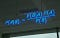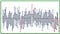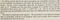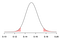# Between Confidence and Credibility

Some researchers confuse frequentist confidence intervals and Bayesian credible intervals.

This article gives an overview of differences between these two intervals.

# Frequentist and Bayesian

The grey of the graph represents the width of the confidence interval, indicating a 95 per cent probability that the true score lies within this area.

This is an incorrect interpretation of confidence intervals, based on a historical schism in statistical practice.

Broadly, there are two schools of thought about what a probability is: frequentist and Bayesians.

Frequentists, as the name suggests, interpret probabilities as the frequencies that events occur in the long run. A fair coin will, at its limit, show Tails half the time: hence, the probability is 1/2.

Bayesians believe probabilities are subjective, reflecting degrees of beliefs about events. Our prior belief is that the probability of a coin showing Tails is 1/2. We flip the coin, updating that belief using Bayes’ Theorem.Bayes’ Theorem is named after Rev Thomas Bayes. (Photo: Matt Buck/Flickr)

# Interval estimation

We use our sample to calculate a statistic, which is an estimate of a population parameter. We can also calculate a range of values — this is called interval estimation.

What is a confidence interval?

In the frequentist framework, the population parameter is fixed but unknown — this parameter does not have a probability distribution.

The sampling distribution of a statistic is its distribution over all possible random samples given that fixed value of the parameter. Frequentist statistical inference is based on the sampling distribution. In short, our parameter is fixed but our data is random.

A 95% confidence interval is an interval — which, when calculated many times — contains the true value of the parameter 95% of the time.As expected, 5 of these 100 confidence intervals do not contain the true population parameter. (Image: Duke University)

Once calculated, we cannot make probabilistic statements about the confidence interval and its containment of the true parameter value. The interval either contains the fixed parameter, or it does not. It is said we are 95% confident that the true value is contained within our calculated interval.Neyman’s original paper on confidence intervals makes this point. (Source: Royal Society)

What is a credible interval?

In the Bayesian framework, we have a subjective prior belief about the population parameter. This parameter is considered to be a variable.

After obtaining the data, our belief is updated: the parameter has the posterior distribution. In short, the data is fixed but the parameter is random.

A 95% credible interval for the parameter is a range of values that has the posterior probability of 95%.The credible interval is an interval of the posterior distribution. (Image: Freakometrics)

By definition, we can make the direct probability interpretation of this interval. However, this definition is not unique — meaning we have to place further constraints to get one interval (such as the highest probability density containing the mode).

There are cases where the calculated intervals are the same, but confidence and credible intervals have radically different interpretations.

Which is better?

It depends on your interpretation of probability and inference.

Frequentist inference is based on answering the question: given an assumed hypothesis, what is the probability we would observe this data?

Bayesian analysis is the reverse: given we have observed this data, what is the probability that the hypothesis holds?

Confidence intervals and credible intervals are sometimes confused, though discussions about frequentist and Bayesian methods are likely to persist.

This blog looks at the use of statistics in Britain and beyond. It is written by RSS Statistical Ambassador and Chartered Statistician @anthonybmasters.

## More from Anthony B. Masters

This blog looks at the use of statistics in Britain and beyond. It is written by RSS Statistical Ambassador and Chartered Statistician @anthonybmasters.